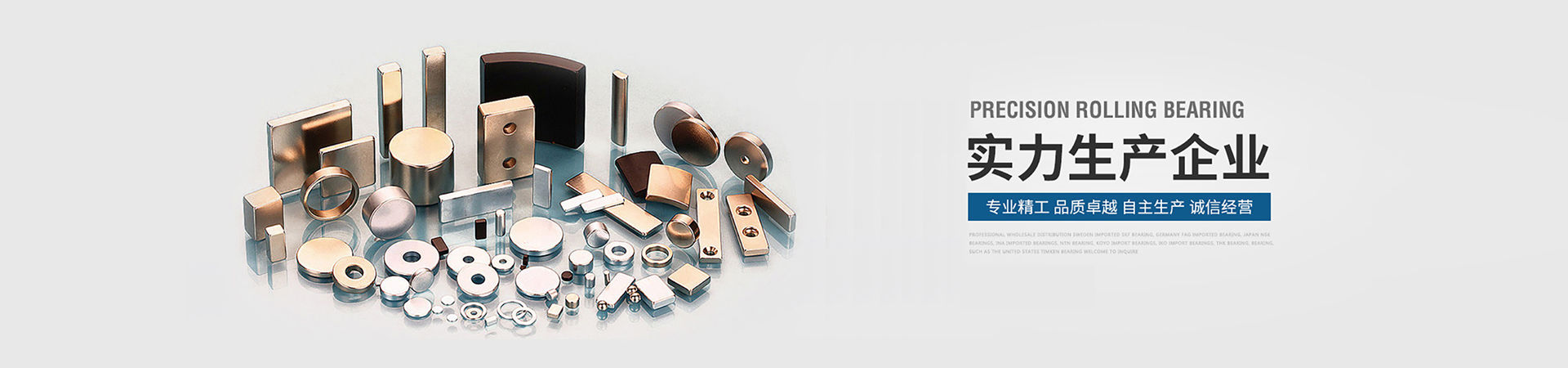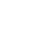Magnetic knowledge

## Magnetic unit common unit conversion

 Magnetic quantity name SI symbols and units CGS symbols and units Unit conversion magnetic flux Φ Weber (Wb) Φ Maxwell (Mx) 1Mx=10-8 Wb Magnetic induction B Tesla (T) B Gauss (Gs) 1Gs=10-4 T Magnetic field strength H An/m (A/m) H Oster 1Oe=103/4p A/m Magnetization M An/m (A/m) M Gauss (Gs) 1Gs=103 A/m Magnetic polarization J Tesla (T) 4pM Gauss (Gs) 1Gs=10-4 T Magnetic energy product BH Coke/m3 (J/m3) BH Gao Ou (GOe) 1MGOe=102/4p kJ/m3 Vacuum permeability4p•10-7H/m - 1 -## Magnetic concept

Permanent magnet material: The permanent magnet material is magnetized by an external magnetic field and does not disappear. It can provide a stable magnetic field to the external space. There are four common metrics for NdFeB permanent magnets:

Remanence (Br) : Units are Tesla (T) and Gauss (Gs) 1Gs = 0.0001T

A magnet is magnetized by an external magnetic field in a closed circuit environment to cancel the external magnetic field after the technology is saturated. At this time, the magnetic induction intensity exhibited by the magnet is called remanence. It represents the maximum flux value that the magnet can provide. It can be seen from the demagnetization curve that it corresponds to the case where the air gap is zero, so the magnetic induction intensity of the magnet in the actual magnetic circuit is smaller than the remanence. NdFeB is the highest practical permanent magnet material found in Br today.

The magnetic coercivity (Hcb) unit is A/m (A/m) and Oe (Oe) or 1 Oe≈ 79.6A/m.
The value of the reverse magnetic field strength required to reduce the magnetic induction to zero when the magnet after the technical saturation magnetization is reversely magnetized is called the magnetic induction coercive force (Hcb). However, the magnetization of the magnet is not zero at this time, but the applied reverse magnetic field and the magnetization of the magnet cancel each other out. (The external magnetic induction performance is zero.) At this time, if the external magnetic field is removed, the magnet still has a certain magnetic property. The coercive force of NdFeB is generally above 11,000 Oe.

The intrinsic coercive force (Hcj) unit is A/m (A/m) and Oe (Oe)1 Oe≈79.6A/m

The magnetization of the magnet is reduced to zero. The magnitude of the applied reverse magnetic field is called the intrinsic coercivity. The intrinsic coercivity is a physical quantity that measures the anti-demagnetization ability of a magnet. If the applied magnetic field is equal to the intrinsic coercivity of the magnet, the magnetism of the magnet will be substantially eliminated. The Hcj of NdFeB will decrease with increasing temperature, so you should choose a high Hcj grade when you need to work in a high temperature environment.

The unit of magnetic energy product (BH) is Coke/m3 (J/m3) or high (GOe) 1 MGOe≈7. 96k J/m3

The product of B and H at any point on the demagnetization curve is both BH we call the magnetic energy product, and the maximum value of B x H is called the maximum energy product (BH)max. The magnetic energy product is one of the important parameters of the energy stored by the constant magnet. The larger the (BH)max, the larger the magnetic energy contained in the magnet. When designing the magnetic circuit, the working point of the magnet should be as close as possible to the B and H corresponding to the maximum magnetic energy product.

Isotropic magnet: A magnet with the same magnetic properties in any direction.

Anisotropic magnets: Magnetic properties vary in different directions; and there is one direction in which the magnet with the highest magnetic properties is obtained. The sintered NdFeB permanent magnet is an anisotropic magnet. Orientation direction: The direction in which an anisotropic magnet can obtain the best magnetic properties is called the orientation direction of the magnet. Also known as "orientation axis", "easy magnetization axis". Magnetic field strength: refers to the size of the magnetic field somewhere in space. It is represented by H, and its unit is ampere/meter (A/m).

Magnetization: refers to the vector sum of magnetic moments per unit volume of the material, expressed in M, in units of ampere/meter (A/m).

Magnetic induction: The magnetic induction B is defined as: B = μ0 (H + M), where H and M are magnetization and magnetic field strength, respectively, and μ0 is vacuum permeability. The magnetic induction is also called the magnetic flux density, that is, the magnetic flux per unit area. The unit is Tesla (T).

Magnetic flux: The total magnetic induction in a given area. When the magnetic induction B is uniformly distributed on the surface A of the magnet, the general formula of the magnetic flux Φ is Φ = B × A. The SI unit of flux is Maxwell.

Relative Permeability : The ratio of the media permeability to the vacuum permeability, ie μr = μ/μo. In the CGS unit system, μo=1. In addition, the relative magnetic permeability of air tends to be 1 in practical use, and the relative magnetic permeability of copper, aluminum and stainless steel materials is also approximately 1.

Permeance: The ratio of flux Φ to magnetomotive force F, similar to the conductance in a circuit. It is a physical quantity that reflects the magnetic permeability of a material.

The permeability coefficient Pc is again the demagnetization coefficient. On the demagnetization curve, the ratio of the magnetic induction intensity Bd to the magnetic field strength Hd, that is, Pc = Bd/Hd, the permeability coefficient can be used to estimate the magnetic flux value under various conditions. For the isolated magnet Pc, it is only related to the size of the magnet. The intersection of the demagnetization curve and the Pc line is the operating point of the magnet. The larger the Pc is, the higher the working point of the magnet is, and the less likely it is to be demagnetized. In general, the larger the orientation length for an isolated magnet, the larger the Pc. Therefore, Pc is an important physical quantity in the design of permanent magnet magnetic circuits.

### 联系 CONTACTS### Service Hotline：

+86-13858970905

Contact：Mr. Shen

Tel：+86-579-86575877

Fax：+86-579-86575833

Email：umagnet@qq.com; scz@u-magnets.com

Address：No. 55, Xianghe Road, electronic industrial park, Hengdian Town, Dongyang City, Zhejiang province